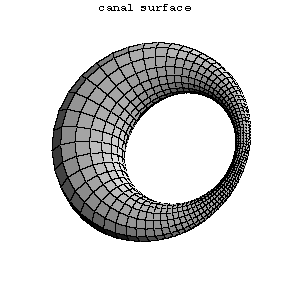# Canal surface

Kanalfläche

A surface for which one family of curvature lines consists of circles; the plane of each such circle intersects the surface along this circle at a constant angle. The corresponding component of the focal set of the canal surface degenerates to a curve $\Gamma$, and hence the canal surface is the envelope of a one-parameter family of spheres having the corresponding principal radii of curvature as their radii. Conversely, if a regular curve $\zeta(s)$ is given, parametrized with arc length $s$, and $R(s)$ is the radius function for a one-parameter family of spheres with centres in $\zeta(s)$, then the canal surface enveloping this family is characterized by the following equations for its radius vector $r$: $$\langle r - \zeta(s), r - \zeta(s) \rangle = R^2(s) \,,$$ $$\langle r - \zeta(s), \zeta'(s) \rangle = -R'(s).R(s)$$ ($\langle,\rangle$ denoting the scalar product in $\mathbf{E}^3$). This equation can be solved if and only if $|R'(s)| \le 1$. If $R = \text{const.}$, then the canal surface is called a tubular surface, for which the torus may serve as an example.